Help Center
1. Help Center
2. Working with Surveys

# Creating a Calculation for a Survey

### Creating a Calculation

Calculations take the results of the Questions and Lookups and allow you to calculate values from those questions. Calculations can also reference the results of other calculations. ScopeStack enables you to add, subtract, multiply, and divide to create values that can be used in Recommendations.

To create a Calculation, you can click + Add Calculation.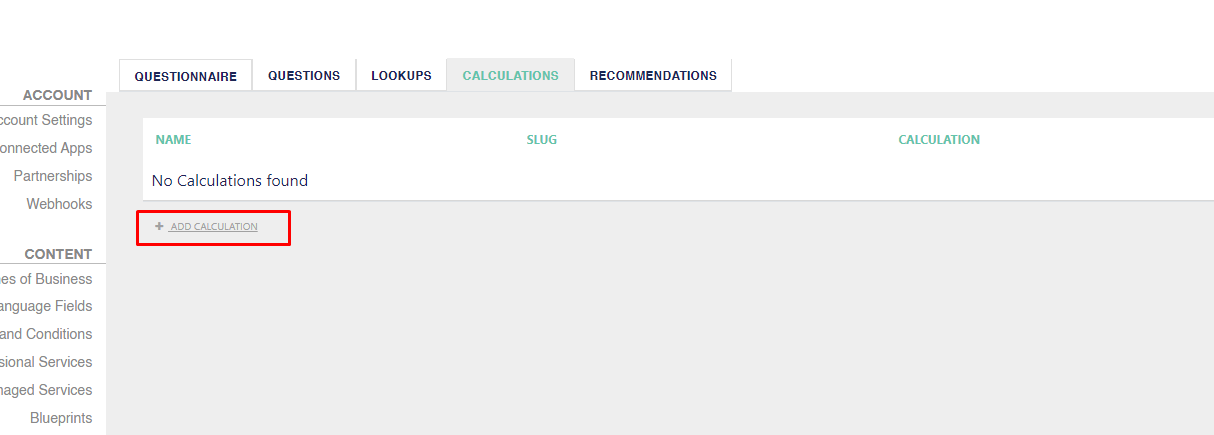If you need to insert a simple value into a recommendation, you can also create a calculation that adds 0 + 1 to return that value.

### Basic Calculations

To create a basic calculation such as a number based on a response to a question, you can use the name of the questions with the available mathematical operands available. These can be found on the right side of the page in a Calculation.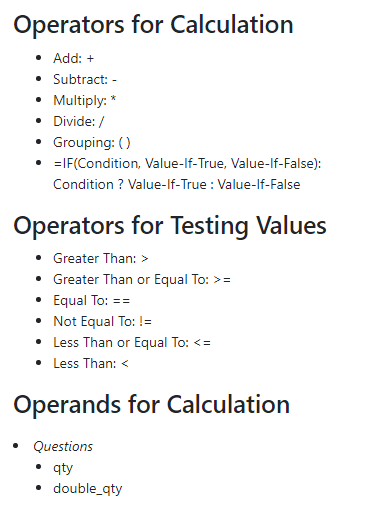In this screenshot, we see an example of a calculation that takes the response to the first question and multiplies it by the response to the second question.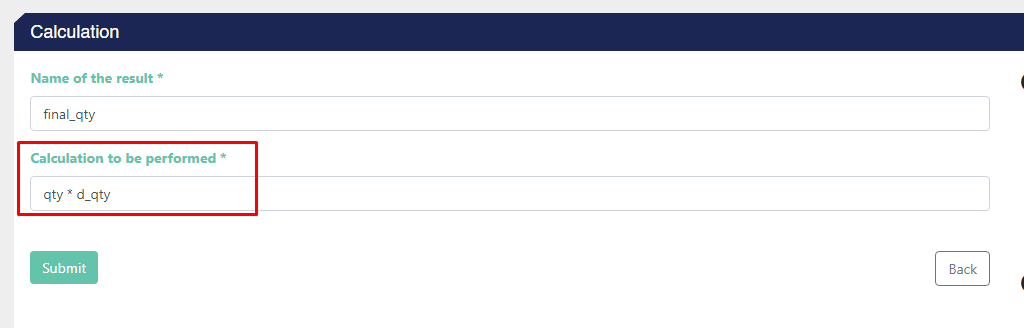You can use this final value to attach to a service in “Recommendations.”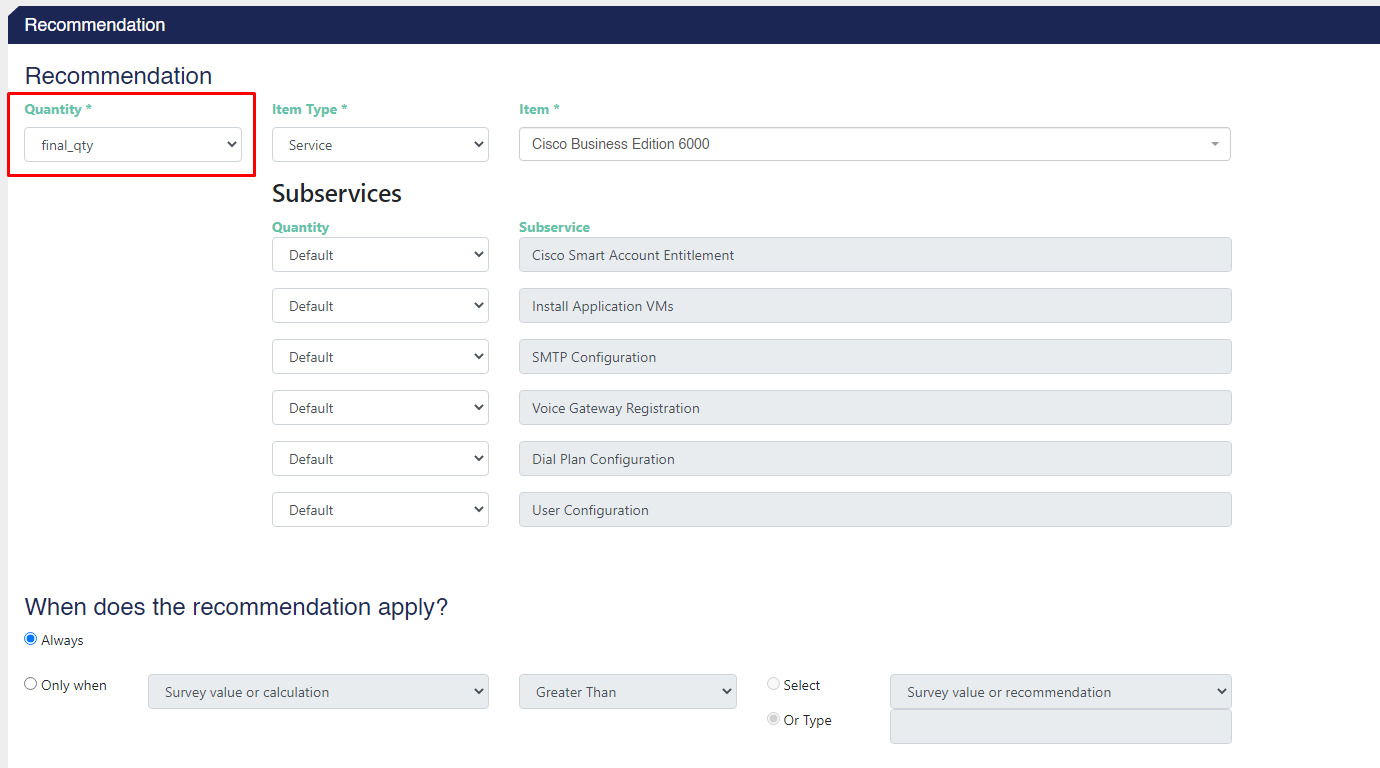### Conditional Statements

With conditional statements, you can make more complex calculations in your survey.

In the screenshot below we can see a conditional statement that checks if the value of the question "qty" is greater than 2. If it is, the calculation returns a value of 100. If it is not then the calculation will return a value of 50.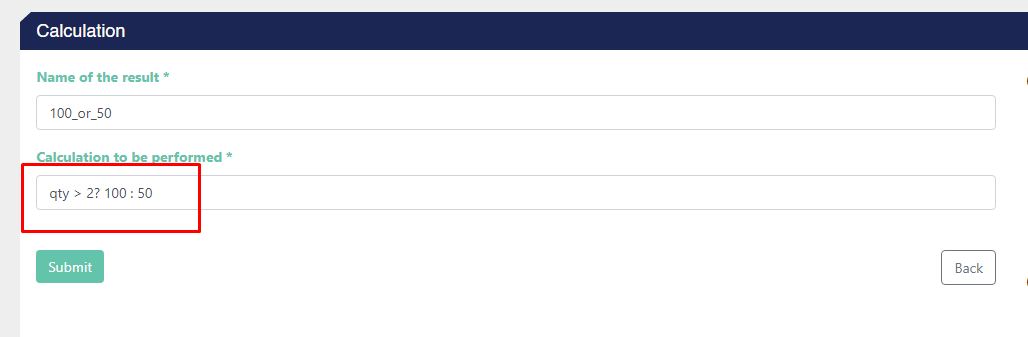In this example, the question mark indicates the condition, the number after indicates the value if the condition is true, and the colon indicates that if the previous statement is not true, the next number will be returned.

In the screenshot below we can see the final result of our survey when we give a quantity greater than 2.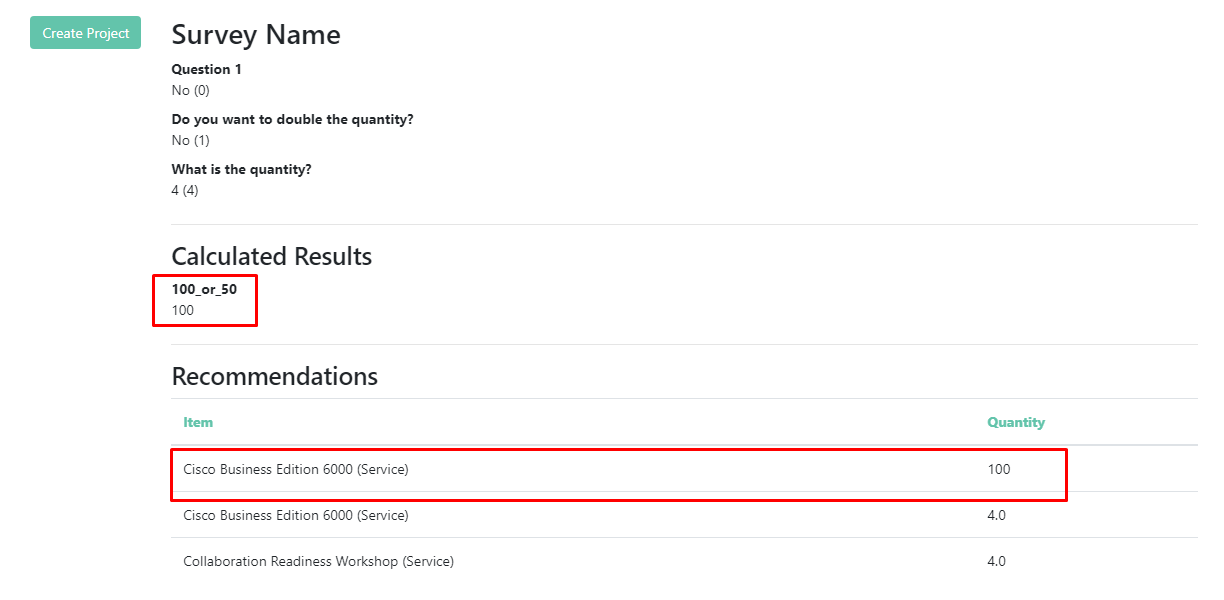Congratulations on making your first calculation for a survey in ScopeStack 🎉